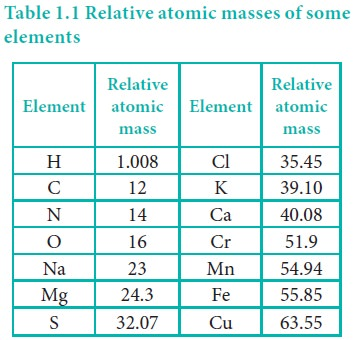Home | | Chemistry 11th std | Molecular Mass

# Molecular Mass

molecular mass is defined as the ratio of the mass of a molecule to the unified atomic mass unit.

Molecular Mass

Similar to relative atomic mass, relative molecular mass is defined as the ratio of the mass of a molecule to the unified atomic mass unit. The relative molecular mass of any compound can be calculated by adding the relative atomic masses of its constituent atoms.

## For example,

i) Relative molecular mass of hydrogen molecule (H2)

= 2 × (relative atomic mass of hydrogen atom)

= 2 × 1.008 u

= 2.016 u.

ii) Relative molecular mass of glucose (C6H12O6)

= (6 × 12) + ( 12 ×1.008) + (6 ×16)

= 72+12.096+96

= 180.096 u

Table 1.1 Relative atomic masses of some elementsStudy Material, Lecturing Notes, Assignment, Reference, Wiki description explanation, brief detail
11th Chemistry : UNIT 1 : Basic Concepts of Chemistry and Chemical Calculations : Molecular Mass |